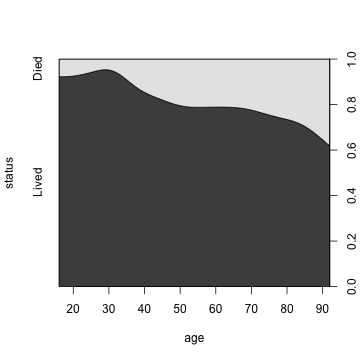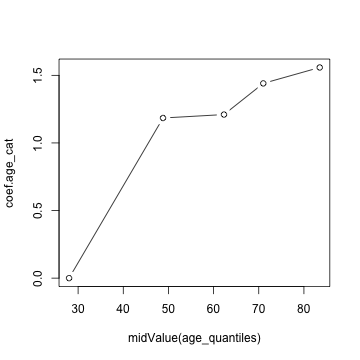# Linearity in logistic regression

``````## Same data available online:
## Hosmer and Lemeshow (2000) Applied Logistic Regression
## http://www.umass.edu/statdata/statdata/data/index.html
## description: http://www.umass.edu/statdata/statdata/data/icu.txt
## text format: http://www.umass.edu/statdata/statdata/data/icu.dat
## Excel format: http://www.umass.edu/statdata/statdata/data/icu.xls

skip = 8, col.names = c("id","status","age","sex","race","service","cancer","renal","inf","cpr","sys","heart","prevad","type","frac","po2","ph","pco2","bic","cre","loc")
)

## Recode variables
icu <- within(icu, {
status  <- factor(status,  levels = 0:1, labels = c("Lived","Died"))
sex     <- factor(sex,     levels = 0:1, labels = c("Male","Female"))
race    <- factor(race,    levels = 1:3, labels = c("White","Black","Other"))
service <- factor(service, levels = 0:1, labels = c("Medical","Surgical"))
type    <- factor(type,    levels = 0:1, labels = c("Elective","Emergency"))
po2     <- factor(po2,     levels = 0:1, labels = c(">60","<=60"))
ph      <- factor(ph,      levels = 0:1, labels = c(">=7.25","<7.25"))
pco2    <- factor(pco2,    levels = 0:1, labels = c("<=45",">45"))
bic     <- factor(bic,     levels = 0:1, labels = c(">=18","<18"))
cre     <- factor(cre,     levels = 0:1, labels = c("<=2.0",">2.0"))
loc     <- factor(loc,     levels = 0:2, labels = c("No Coma or Deep Stupor","Deep Stupor","Coma"))
})

## Recode No-Yes variables

icu[,no.yes.variables] <-
lapply(icu[,no.yes.variables],
function(var) {
var <- factor(var, levels = 0:1, labels = c("No","Yes"))
})

## summary(icu)
``````

## Plot distribution

``````cdplot(status ~ age, icu)
``````## Create categorical age variable based on quantiles (5 groups)

``````age_quantiles <- quantile(icu\$age, 0:5/5)
icu\$age_cat <- cut(icu\$age, breaks = age_quantiles, include.lowest = TRUE)
table(icu\$age_cat)
``````
``````
[16,40] (40,57.6] (57.6,67]   (67,75]   (75,92]
41        39        43        44        33
``````

## Fit models with age as it is and categorical age

If the age variable is used as a continuous variable, the effect of age is uniform across the range of the variable. If it is transformed into a categorical variable, it can have different effects depending on the position in the distribution of the variable.

One effect for age variable

``````## Fit age model (linearity assumption)
logit.age <- glm(status ~ age, icu, family = binomial)
coef(logit.age)
``````
``````(Intercept)         age
-3.05851     0.02754
``````

Three different effect for 1st vs 2nd, 1st vs 3rd, and 1st vs 4th age quantiles

``````## Fit categorical age model
logit.age_cat <- glm(status ~ age_cat, icu, family = binomial)
coef(logit.age_cat)
``````
``````     (Intercept) age_cat(40,57.6] age_cat(57.6,67]   age_cat(67,75]   age_cat(75,92]
-2.539            1.184            1.210            1.440            1.558
``````

## Plot the coefficients against age

``````## Extract coefficients for categorical age variable. Add 0 for reference level.
coef.age_cat <- c(0, coef(logit.age_cat)[-1])
coef.age_cat
``````
``````                 age_cat(40,57.6] age_cat(57.6,67]   age_cat(67,75]   age_cat(75,92]
0.000            1.184            1.210            1.440            1.558
``````
``````
midValue <- function(x) {
x <- (x[-1] + x[-1 * length(x)]) / 2
names(x) <- NULL
x
}

## Get mid values of each quartile
midValue(age_quantiles)
``````
`````` 28.0 48.8 62.3 71.0 83.5
``````
``````
## Plot coefficients against each mid values
plot(y = coef.age_cat, x = midValue(age_quantiles), type = "b")
``````